# KS1 Maths Quiz - Year 1 Shapes - 3D (Questions)

This quiz addresses the requirements of the National Curriculum KS1 Maths and Numeracy for children aged 5 and 6 in year 1. Specifically this quiz is aimed at the section dealing with recognising and naming common 3-D shapes.

Year 1 children will learn to recognise and name basic and common 3-D shapes such as cuboids, pyramids and spheres. They will use 3-dimensional shapes when building and be encouraged to recognise, spot and name these common shapes in the real world.

To see a larger image, click on the picture.
1. This shape is a...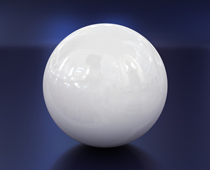[ ] Pyramid [ ] Cuboid [ ] Cube [ ] Sphere
2. This shape is a...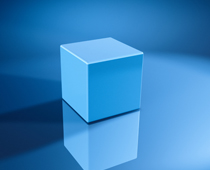[ ] Square [ ] Pyramid [ ] Sphere [ ] Cube
3. There are lots of _______ and 1 _______ in this picture.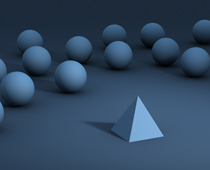[ ] Spheres, pyramid [ ] Circles, triangle [ ] Spheres, cuboid [ ] Spheres, triangle
4. This is a _______ made up of _____.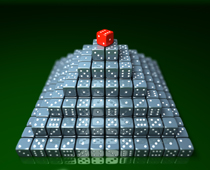[ ] Triangle, squares [ ] Tower, circles [ ] Pyramid, spheres [ ] Pyramid, cubes
5. These are called sugar...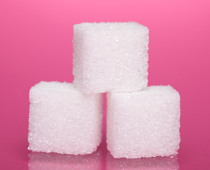[ ] Spheres [ ] Squares [ ] Pyramids [ ] Cubes
6. Why are balls used in sports usually spheres?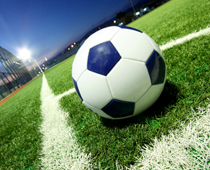[ ] They look better [ ] Their shape means they roll and bounce well [ ] It's a popular shape [ ] So they can be stored easily
7. These boxes are all...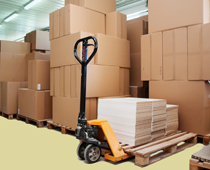[ ] Squares [ ] Cuboids [ ] Spheres [ ] Pyramids
8. This is a ______ made up from _______.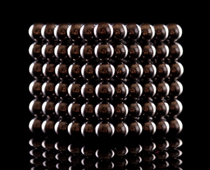[ ] Square, circles [ ] Cuboid, spheres [ ] Cuboid, pyramids [ ] Square, spheres
9. This is a _______ made up of _______.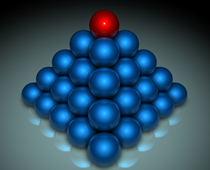[ ] Pyramid, cubes [ ] Pyramid, oblongs [ ] Triangle, circles [ ] Pyramid, spheres
10. A cube is also a...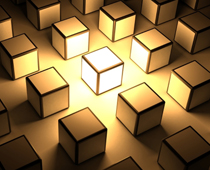[ ] Square [ ] Pyramid [ ] Sphere [ ] CuboidKS1 Maths Quiz - Year 1 Shapes - 3D (Answers)
1. This shape is a...[ ] Pyramid [ ] Cuboid [ ] Cube [x] Sphere
The ball is completely round and is a sphere
2. This shape is a...[ ] Square [ ] Pyramid [ ] Sphere [x] Cube
All its sides and faces are equal
3. There are lots of _______ and 1 _______ in this picture.[x] Spheres, pyramid [ ] Circles, triangle [ ] Spheres, cuboid [ ] Spheres, triangle
There are several spheres but only one pyramid
4. This is a _______ made up of _____.[ ] Triangle, squares [ ] Tower, circles [ ] Pyramid, spheres [x] Pyramid, cubes
The dice are cubes and are stacked up in a pyramid formation
5. These are called sugar...[ ] Spheres [ ] Squares [ ] Pyramids [x] Cubes
Sugar cubes stack well inside a box!
6. Why are balls used in sports usually spheres?[ ] They look better [x] Their shape means they roll and bounce well [ ] It's a popular shape [ ] So they can be stored easily
A sphere rolls well and bounces evenly
7. These boxes are all...[ ] Squares [x] Cuboids [ ] Spheres [ ] Pyramids
Although they are different shapes and sizes, they are all cuboids
8. This is a ______ made up from _______.[ ] Square, circles [x] Cuboid, spheres [ ] Cuboid, pyramids [ ] Square, spheres
This cuboid is made up entirely of spheres!
9. This is a _______ made up of _______.[ ] Pyramid, cubes [ ] Pyramid, oblongs [ ] Triangle, circles [x] Pyramid, spheres
This pyramid is made from spheres stacked in layers
10. A cube is also a...[ ] Square [ ] Pyramid [ ] Sphere [x] Cuboid
Cuboids have 6 faces and 12 edges